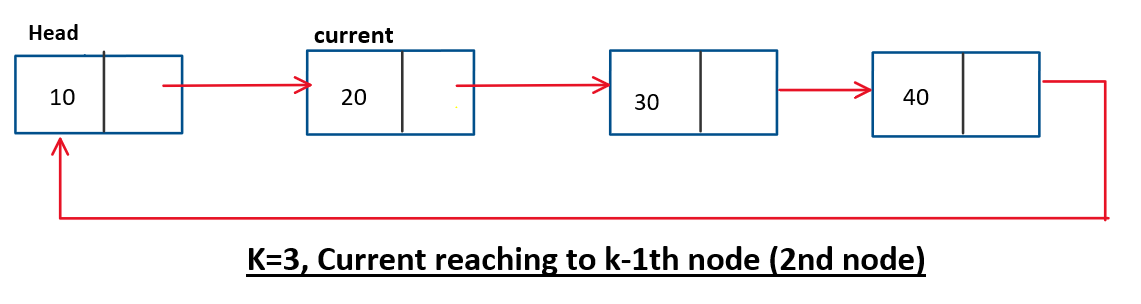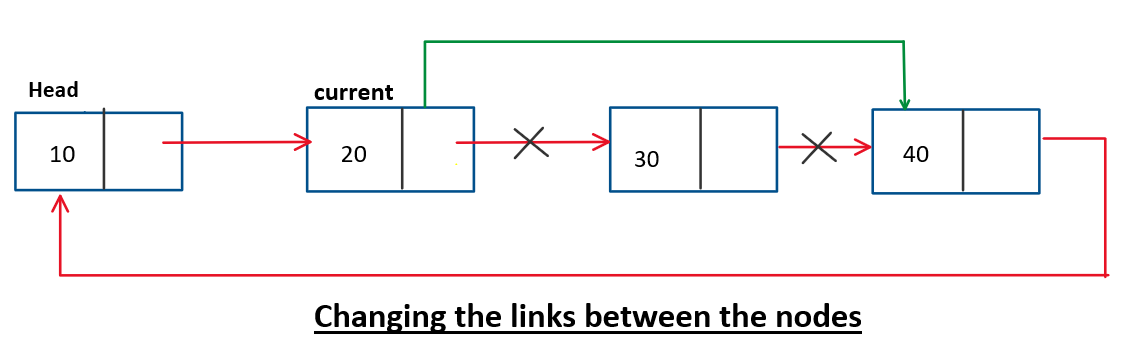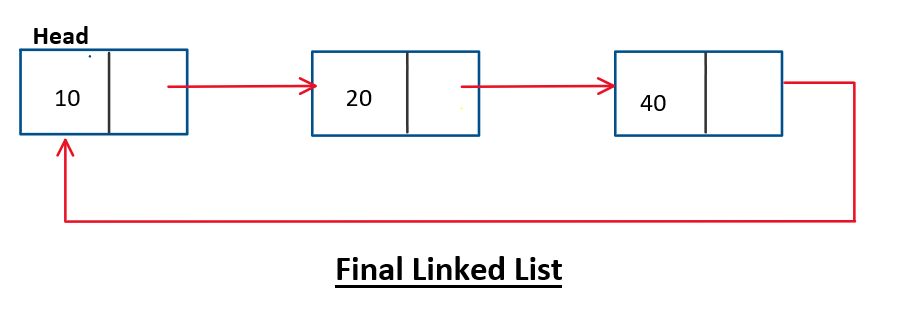# Delete kth node of circular linked list

Problem Statement: Given a circular linked list, delete the kth node of the circular linked list.

Example:

```Example 1:
Input: 2 3 4 5,  k=2
Output: 2 4 5

Example 2:
Input: 1 5 7 9 4, k=4
Output: 1 5 7 4```

### Solution:

DisclaimerDon’t jump directly to the solution, try it out yourself first.

### Approach:

Let’s understand the approach for this problem:

1. Simply iterate to the k-1th node.
2. Store the kth node in a temporary node.
3. Change the next pointer of the k-1th node to point to the k+1th node.
4. Delete the temporary node.
5. Return the head of the linked list.

Here we can handle the case where k=1 which means deleting the head of the linked list. For this, we implement the code for deleting the head of a circular linked list.

The following diagram explains the approachCode:

## C++ Code

``````#include <iostream>
using namespace std;

struct node {
int data;
node * next;
node(int d) {
data = d;
next = NULL;
}
};
node * deleteK(node * head, int k) {
if (head == NULL) return NULL;
if (k == 1) {
head -> data = head -> next -> data;
node * temp = head -> next;
head -> next = head -> next -> next;
delete temp;
}
node * current = head;
for (int i = 0; i < k - 2; i++) {
current = current -> next;
}
node * temp = current -> next;
current -> next = current -> next -> next;
delete temp;
}
void printCll(node * head) {
node * current = head;
do {
cout << current -> data << " ";
current = current -> next;
} while (current != head);
cout<<endl;
}
int main() {
node * head = new node(10);
head -> next = new node(20);
head -> next -> next = new node(30);
head -> next -> next -> next = new node(40);
head -> next -> next -> next -> next = head;
cout<<"Before deleting the kth node in the circular linked list"<<endl;
cout<<"After deleting the kth node in the circular linked list"<<endl;
}
``````

Output:

Before deleting the kth node in the circular linked list
10 20 30 40
After deleting the kth node in the circular linked list
10 20 40

## Java Code

``````class node {
int data;
node next;
node(int d) {
data = d;
next = null;
}
}

public class Main {
public static node deletionK(node head, int k) {
if (head == null) return null;
if (k == 1) {
}
node current = head;
for (int i = 0; i < k - 2; i++) {
current = current.next;
}

current.next = current.next.next;
}
public static void printCll(node head) {
node current = head;
do {
System.out.print(current.data + " ");
current = current.next;
} while (current != head);
System.out.println();
}
public static void main(String args[]) {
node head = new node(10);
head.next = new node(20);
head.next.next = new node(30);
head.next.next.next = new node(40);
System.out.println("Before deleting the kth node in the circular linked list");
System.out.println("After deleting the kth node in the circular linked list");